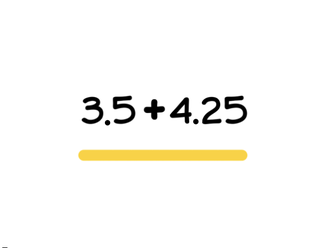Addition with decimal numbers up to the hundredths place

# Addition with decimal numbers up to the hundredths place

Students learn to add decimal numbers up to the hundredths place.8,000 schools use Gynzy92,000 teachers use Gynzy1,600,000 students use Gynzy

## General

Students learn to add decimal numbers up to the hundredths place, they also learn to solve story problems involving decimal numbers.

## Standards

CCSS.MATH.CONTENT.5.NBT.B.

## Learning objective

Students will be able to add decimal numbers up to the hundredths place.

## Introduction

Students practice placing the decimal numbers on the number line. They do so by drawing a line from the decimal number to where it belongs on the number line.

## Instruction

Check that students are able to add decimal numbers up to the hundredths place by asking the following questions:
- What are the steps to solving a story problem?
- What is one way to solve adding decimals?
- How would you add 4.13 + 0.55?

## Quiz

Students are given a set of 10 questions in which they must add decimal numbers up to the hundredths place. The problems are abstract and story problems.

## Closing

Remind students the importance of being able to add decimal numbers up to the hundredths place. Either individually or as a class, ask students to solve the given addition problems. Have students explain their strategy. To close the lesson, students must pick a character. They then leap with the character to an arrow on the number line. Then they leap again. They must add the total of their fist and second leaps. (Example: I select the frog. My first leap is to 0.41 and my second is to 33.21, I add them together for a total of 33.62)

## Teaching tips

Students who have difficulty with this learning goal can be supported by making use of a number line. By representing the addition on the number line it can help make the decimal numbers more tangible.

## Instruction materials

Optional: number line

### The online teaching platform for interactive whiteboards and displays in schools

• Save time building lessons

• Manage the classroom more efficiently

• Increase student engagement